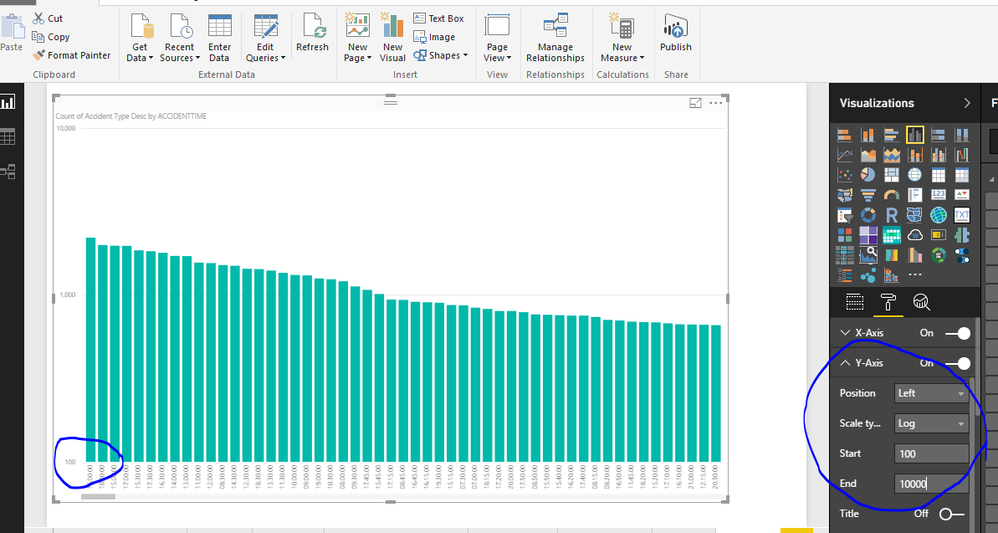cancel
Showing results for
Did you mean:Frequent Visitor

## indexed time series

Hi All

I'm a complete newbie to DAX, while much more experienced with VBA and MySQL. I'm working with percentage growth time series data, sequentially stored in a table by Identifier, Date and Return. Return is transformed from a percentage figure to a factor in the SQL query already (Return/100+1 As Return). To create and indexed time series chart I have entered the following DAX code as a measure:

ReturnTmp =

CALCULATE (

PRODUCT ( Table1[Return] ) * 100 ;

FILTER ( ALL ( Table1 ); Table1[Date] <= MAX ( Table1[Date] ) );

VALUES ( Table1[Identifier] )

)

A - This works fine, but I'm struggling to have the first Return value in the chart set to 100 while all following returns in the time series remain as calculated above. I've tried several approaches with IF , IF &&, and COUNTROW statements, but no success.

B - Once the measure works I have to include it into the final measure by:

ReturnCum =

AVERAGEX ( Table1;Table1[ReturnTmp])

As I understood, there is not possibility to have it in one single measure. Is this correct?

Thank you very much for help.

1 ACCEPTED SOLUTIONFrequent Visitor

Anybody who's interested, here's the solution after a few hours of try and error:

ReturnTmp =
CALCULATE (
IF ( COUNTROWS ( Table1 ) = 1  ;  100  ;  PRODUCT ( Table1 [Return]  ) * 100  ) ;
FILTER ( ALL ( Table1 ) ;  Table1[Date] <= MAX (Table1[Date]  )  )  ;
VALUES ( Table1[Identifier] )
)

This is a rather standard chart in financial analysis for plotting return series.

5 REPLIES 5Community Champion

For Question A

See the screenshot, You can adjust the settings shown in the screenshot in Analytics pane.For Question B

Thanks & Regards,
Bhavesh

Love the Self Service BI.Frequent Visitor

Hi Bavesh

Thank you very much for your reply. I will work through the blog for question B.

The proposed solution for A would only set the crossing point of the x-Axis to the y-Axis at 100 and not rebase the time series themselves to 100. See this screenshot, I need the series all to start at 100 for correct comparability of the graphs in the chart.Thank you very much for any help on this.

OttoCommunity Support

Hi @OCK,

If I understand you correctly, you want to show the first point for all series which are around 100 in the line chart, right?

If that is a case, assume in 2004 year, all series data point are around 100. Then you can drag the X-axis field into the Visual level filter, set the "is greater than or equal to" 2004.But if in 2004 year, only 1 series data point around 100, while other series's data point are around 100 in 2008 year. In this scenario, we can't move those series to the left side of the chart, to display on 2004 year. As in the chart, X-axis and Legend are groups, the position data points values display is based on the intersection of the X-axis and Legend.

Best Regards,
Qiuyun Yu

Community Support Team _ Qiuyun Yu
If this post helps, then please consider Accept it as the solution to help the other members find it more quickly.Frequent Visitor

Hi Qiuyun Yu,

Thank you very much for your reply. Unfortunately this cannot be done with filtering data on the visual, it must be set in the DAX function. The first value in the series must be set to 100 while all following items will apply the formula as outline above. Hence it must be something around this:

IF (Table1[Date] = MIN ( Table1[Date] ) ; 100 ; PRODUCT ( Table1[Return] ) * 100

With this filter applied:

FILTER ( ALL ( Table1 ); Table1[Date] <= MAX ( Table1[Date] ) );

VALUES ( Table1[Identifier] )

Thank you,

OCKFrequent Visitor

Anybody who's interested, here's the solution after a few hours of try and error:

ReturnTmp =
CALCULATE (
IF ( COUNTROWS ( Table1 ) = 1  ;  100  ;  PRODUCT ( Table1 [Return]  ) * 100  ) ;
FILTER ( ALL ( Table1 ) ;  Table1[Date] <= MAX (Table1[Date]  )  )  ;
VALUES ( Table1[Identifier] )
)

This is a rather standard chart in financial analysis for plotting return series.